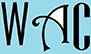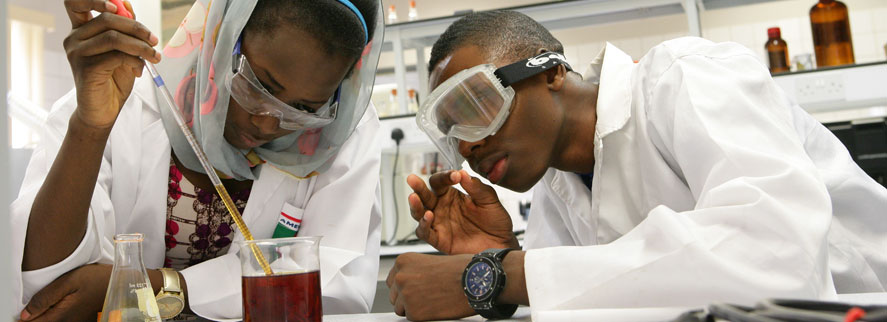## Geometry Question for Title 1 Schools: Circumference Question?

2) What is the circumference of a circle with a diameter of 8?

Circumference = 2 пr, where п = 3.14and r = radius (half of a diameter “D”)

If D = 8, then r = 4

Circumference = 2 x 3.14 x 4

25.12